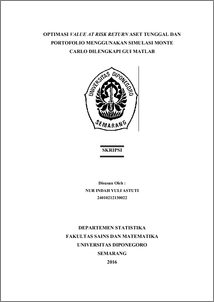# OPTIMASI VALUE AT RISK RETURN ASET TUNGGAL DAN PORTOFOLIO MENGGUNAKAN SIMULASI MONTE CARLO DILENGKAPI GUI MATLAB

ASTUTI, NUR INDAH YULI (2016) OPTIMASI VALUE AT RISK RETURN ASET TUNGGAL DAN PORTOFOLIO MENGGUNAKAN SIMULASI MONTE CARLO DILENGKAPI GUI MATLAB. Undergraduate thesis, Fakultas Sains dan Matematika, Undip.Preview
PDF
4Mb

## Abstract

Matlab (Matrix Laboratory) is an interactive programming system with the basic elements of array database which dimensions do not need to be stated in particular, while the GUI is the submenu of Matlab. Matlab GUI generally more easily learned and used because in worked it does not need to know the commandments and how command works. Value at Risk (VaR) is a scale that can measure the maximum loss that may happen for a specified period of time in the normal market conditions at a certain level of confidence. VaR also can be used to specify investor’s target. The most important thing in the VaR is to determine the type of methodology and assuming appropriate with the distribution of the return. One of the methods in calculating the VaR is Monte Carlo simulation. VaR with Monte Carlo simulation method assumes that the return value is normal distribution simulated using the appropriate parameters and portfolio return is linier towards its single asset return. From the results and analysis research conducted use GUI Matlab, VaR single asset of value risk on the stock of United Tractors Tbk (UNTR) is greater than Bank Rakyat Indonesia (Persero) Tbk (BBRI), Astra International Tbk (ASII), and Bank Negara Indonesia Tbk ( BBNI), VaR value of portfolio consisting of two assets, the three assets, and four assets have lower value than the sum of its single asset of the value of VaR. In this research, determining the level of trust and specified time period is very important to count of VaR value because it can describe how much investors bear the risk. Keywords: Matlab GUI, Value at Risk, time period, confidence level, Monte Carlo simulation.

Item Type: Thesis (Undergraduate) H Social Sciences > HA Statistics Faculty of Science and Mathematics > Department of Statistics 55038 Mr Hasbi Yasin 25 Jul 2017 14:01 25 Jul 2017 14:01

Repository Staff Only: item control page